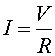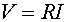Ohm's law A resistor does exactly that. It resists current. Larger resistances resist current more. For a voltage V applied across the ends of a resistor, the current I through the resistor is:An easy way to remember this equation is "Vermont = Rhode Island",. R is known as the resistance. It is in units of ohms which are equivalent to volts per amp.